# Magnetic Properties of Coordination Complexes Notes | Study Inorganic Chemistry - Chemistry

## Chemistry: Magnetic Properties of Coordination Complexes Notes | Study Inorganic Chemistry - Chemistry

The document Magnetic Properties of Coordination Complexes Notes | Study Inorganic Chemistry - Chemistry is a part of the Chemistry Course Inorganic Chemistry.
All you need of Chemistry at this link: Chemistry

MAGNETIC PROPERTIES

When a substance is placed in a magnetic field, the intensity of the magnetic field may increase or decrease depending upon the substance. There are five types of magnetism:

1. Paramagnetism: It is a property of substances which are attracted in a magnetic field.
Paramagnetism derives from the spin and orbital angular momenta of electrons. When all the electrons in a molecule are paired, their spin and orbital angular momenta cancel each other. Paramagnetism arises as a result of unpaired electron spins in the atom. Such substances are said to be paramagnetic. When a paramagnetic substance is placed in an external magnetic field, the permanent magnetic moment tends to align themselves in the direction of external magnetic field. In the absence of a magnetic field, the magnetic moments of individual molecule are randomly distributed. Hence, no magnetic moment is observed in the absence of magnetic field. Paramagnetism also depends upon temperature.

2. Diamagnetism: The substances having only paired electrons exhibit a weak magnetic moment known as diamagnetism and such substances are called diamagnetic substances. Diamagnetic substances are repelled by magnetic field. Diamagnetism is independent of temperature and the effect is much weaker than Paramagnetism. Magnetic permeability P is less than 1 for diamagnetic substances.
When the paramagnetic species are very close together (as in the bulk metal) or are separated by a species that can transmit magnetic interactions (as in many d-block metal oxides, fluorides and chlorides), the metal centres may interact (couple) with one another. The interaction may give rise to ferromagnetism or antiferromagnetism.

3. Ferromagnetism: In a ferromagnetic material, large domains of magnetic dipoles are aligned in the same direction. Ferromagnetism is actually greatly enhanced Paramagnetism due to close alignment of magnetic dipoles in the same direction.

4. Antiferromagnetism: Antiferromagnetism arises when the magnetic moments at neighbouring centres are aligned in opposite directions. The classic example of antiferromagnetism is MnO.

5. Ferrimagnetism: Ferrimagnetism is shown by substances in which the individual magnetic moments are aligned so as to oppose others but giving rise to some finite resultant magnetic moment. For example, Fe3O4 is ferromagnetic.
The alignment of individual magnetic moments in paramagnetic, ferromagnetic, antiferromagnetic and ferromagnetic substances is shown below: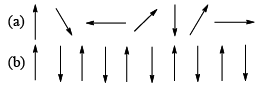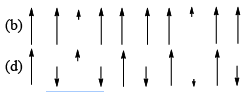(a) Paramagnetism, (b) ferromagnetism, (c) antiferromagnetism and (d) ferrimagnetism

Superechange: When a bridging ligand facilitates the coupling of electron spins on adjacentmetal centres, the mechanism is one of superexchange. In a superexchange pathway, the unpaired electron on the first metal centre, M1, interacts with a spin-paired pair of electrons on the bridging liga nd wit h the result that the unpaired electron on M2 is aligned in an antiparallel manner wit h respect to that on M1.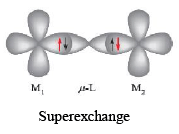Magnetic Susceptibility: Magnetic moment is calculated from the measurement of magnetic susceptibilit y. T herefore we will first discuss how to calculate magnetic susceptibilit y and then will move to the magnetic moment.
When a material is placed in a magnetic field of strength H, the induced magnetic field (B) produced in the substance will either be greater or less than the applied magnetic field depending upon whether the substance is paramagnetic or diamagnetic. The difference between the induced magnetic field (B) and applied magnetic field (H) is given by,
∆H = B – H
The magnetic susceptibilit y obtained experimentally is the specific (or mass) susceptibilit y χ(chi) which is given by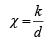Where κ is the magnetic susceptibilit y per unit volume of the substance and is called volume magnetic susceptibilit y. The κ is the measure of the extent to which a substance tends to be magnetized. d is the density of the sample.
Molar susceptibilit y χM is obtained by multiplying χ by the molecular mass of the sample.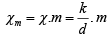Molar susceptibility is a measure of the degree to which one mole of substance of substance interacts with the applied magnetic field. Paramagnetic susceptibility is much greater than a diamagnetic substance.
Molar susceptibility is related to the magnetic moment of the substance according to equation (if small diamagnetic contribution is removed):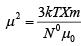Where, Where k = Bo ltzmann constant (1.38 × 10–23 Jk–1)
μ0 → permeabilit y of free space (4 π × 10–7 Hm–1)
T = absolute temperature, N0 = Avogadro’s constant μ has SI unit → JT–1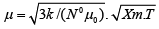μ  is expressed in Bohr magnetons (μB) where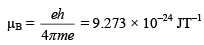where e is electronic charge, h is Plank’s constant and me is mass of an e
Thus magnetic moment in Bohr magnetons is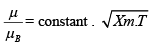or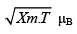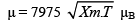Magnetic Moment: The unpaired electron gives rise to a magnetic field because of its spin, and also because of the orbitals angular momentum. The general equation for the magnetic moments of the first row transition metal ions is:

μ (S + L) =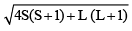Where S is the total of spin quantum numbers and L is the resultant of the orbital angular momentum quantum numbers of all the electrons in the molecule.

For an electron the spin quantum no. ms is ±1/2, hence

S = ms . n

Where n is the no. of unpaired electrons.
In many compounds of the first row transition elements, the orbital contribution is quenched by the electric fields of surrounding atoms. If we ignore the orbital contribution, the observed magnetic moment is spin only magnetic moment μs and is given by,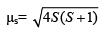Since S = ms . n, the magnetic moment is related to no. of unpaired electron by equation: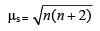The spin only magnetic moment formula gives good agreement with many high spin complexes of first row elements. This is given in the following table.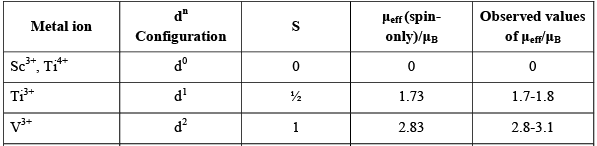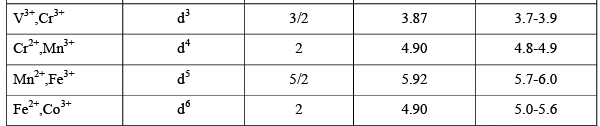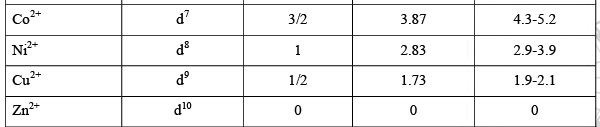But there are exceptions in some cases. For example, for Co2+, the observed value for magnetic moment is higher than calculated by the spin only formula. This suggests there is also an orbital contribution.

Orbital Contribution

To have an orbital contribution it must be possible to transform an orbital into an equivalent (degenerate) orbital by rotation. It is possible to transform t2g orbitals (dxy, dyz, dzx) into each other by rotating 90˚. It is not possible to transform the eg orbitals (dx2-y2 and dz2) in this way since the y have different shapes. Therefore these orbitals have no orbital contribution. If all the t2g orbitals are singl y occupied, then it is not possible to transform the dxy into dyz or dzx since t he y already contain an electron wit h same spin. Similarl y it is not possible to transform the t2g orbitals if t he y are all doubly occupied. Thus configurations with (t2g)3 or (t2g)6 have no orbital contribution, but the others all have an orbital contribution. Therefore, in octahedral complexes following arrangements have an orbital contribution: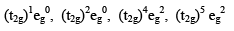has (t2g)5(eg)2 configuration: hence the high value of magnetic moment is due to orbital contribution. Similarly, an orbital contribution arises in tetrahedral complexes with the following configurations: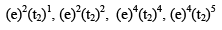In the second and third row transition elements, and particularly in the lanthanide elements, the orbital motion is not prevented or quenched. Thus the orbital contribution L is included in the calculations. In some cases there is coupling between the spin contribution S and an angular contribution L (spin-orbit coupling or Russel-Saunders coupling) to give a new quantum no. J. In this case the magnetic moment is given by,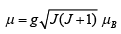Where g is the gyromagnetic ratio and is given as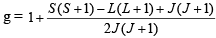on rearranging, this becomes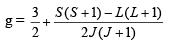Spin orbit coupling gives rise to fine structure in the absorption spectra. It splits the lower levels into a set of different levels. These levels may be populated by using thermal energy, giving rise to a temperature dependent magnetic moment.

Effect of Temperature on Magnetic Moment: According to Curie’s Law paramagnetic susceptibility χM varies inversely with absolute temperature.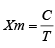Where C is a Constant characteristic for the particular substance and is called Curie’s constant. Thus the moment in Bohr’s magnetons is given by,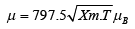The Curie Law is valid only for paramagnetic substances which are magnetically dilute. In some substances which are not magnetically dilute, the magnetic moments of unpaired electrons on neighbouring atoms may couple with each other. These substances behave as ferromagnetic, antiferromagnetic or ferrimagnetic and obey Curie- Weiss Law: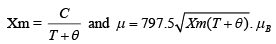θ is called Weiss constant and is an empirical quantit y.
The temperature above which any ferromagnetic substance behaves as normal paramagnetic substance is called Curie temperature (TC). In case of ant i-ferromagnetic substances the magnetic susceptibilit y increases with temperature initially and then decreases. The temperature at which the anti-ferromagnetic substances start behaving as paramagnetic substances is called Neel Temperature (TN).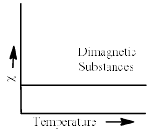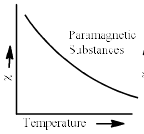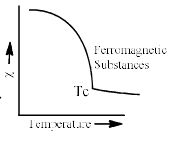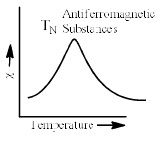Spin Crossover: Octahedral complexes of d4, d5, d6 and d7 of first transition metal ions can eit her be high spin or low spin depending upon the magnitude of crystal ∆o and pairing energy P. When magnitude of ∆o ≈ P, the two complexes (HS and LS) have same energy and they exist in equilibrium. Spin crossover can occurs in these complexes by temperature or pressure.
For example, the complex [Fe(CN)5(NH3)]3- undergoes spin crossover (LS to HS) b y applying pressure.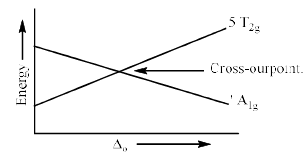The most important examples in which spin crossover take place are [Fe(Phen)2(NCS-N)2] and [Fe(btz)2(NCS-N)2]. At high temperature [Fe(Phen)2(NCS-N)2] exists as high spin with four unpaired electrons. Hence magnetic moment is more. When the temperature is decreased, there is sharp decrease in magnetic moment at 175K and the complex becomes low spin. This indicates that the complex [Fe(Phen)2(NCS-N)2] exist as both high spin and low spin at 175K. In [Fe(btz)2(NCS-N)2] low- to highspin crossover occurs more gradually. The dependence of the magnetic moment on temperature for these complexes are plotted below: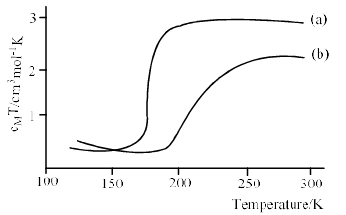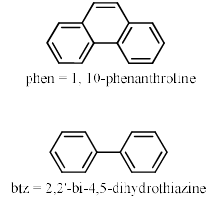(a) [Fe(Phen)2(NCS-N)2]                                                 (b) [Fe(btz)2(NCS-N)2]

The document Magnetic Properties of Coordination Complexes Notes | Study Inorganic Chemistry - Chemistry is a part of the Chemistry Course Inorganic Chemistry.
All you need of Chemistry at this link: ChemistryUse Code STAYHOME200 and get INR 200 additional OFF

## Inorganic Chemistry

49 videos|71 docs|16 tests

Track your progress, build streaks, highlight & save important lessons and more!

,

,

,

,

,

,

,

,

,

,

,

,

,

,

,

,

,

,

,

,

,

;